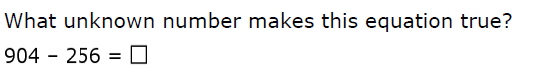Name *
1.
1 point2. Does replacing the unknown with 7 make each equation true? Select ALL answers that make this true.
1 point
3.
1 point4.
1 point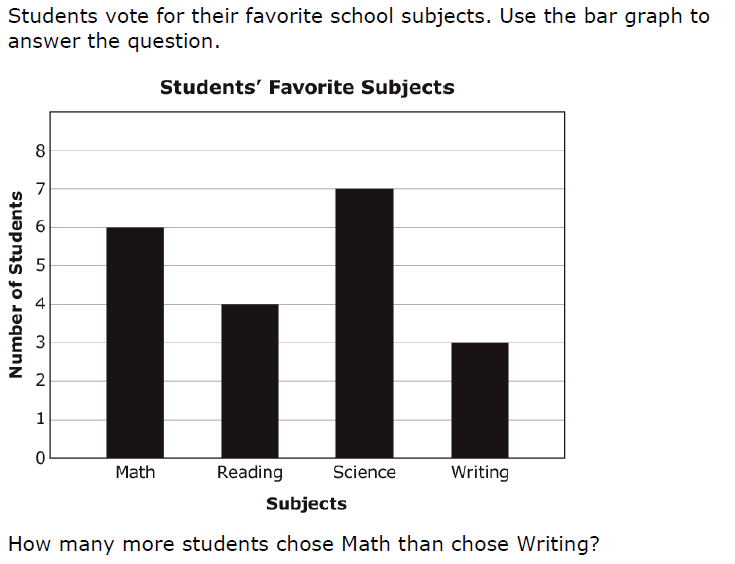5.
2 points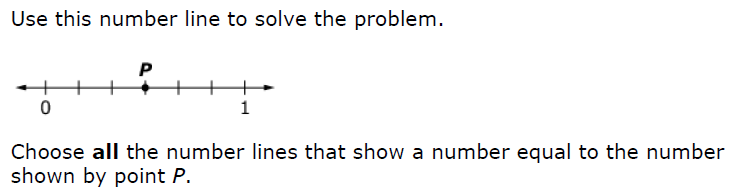6.
1 point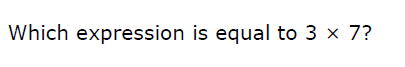7.
0 points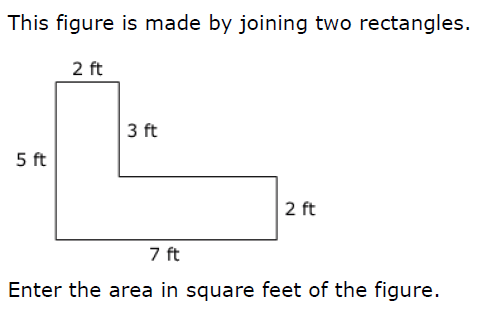8.
1 point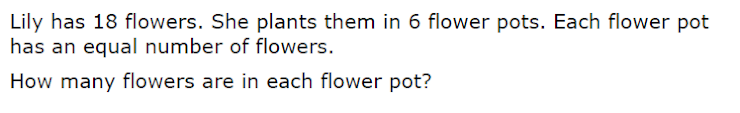9.
1 point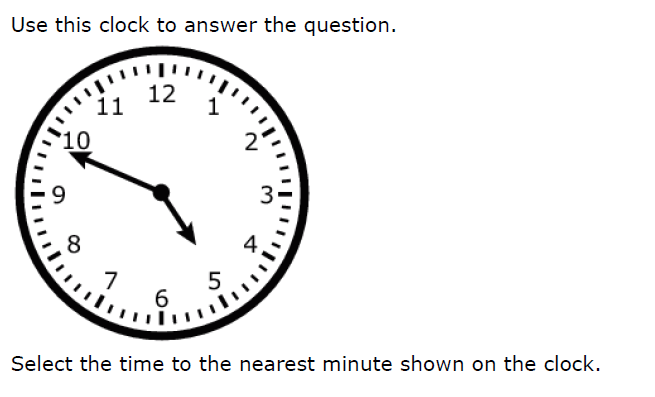10. Decide if each equation is True or False. Select all of the equations that are true.
1 point
11.
1 point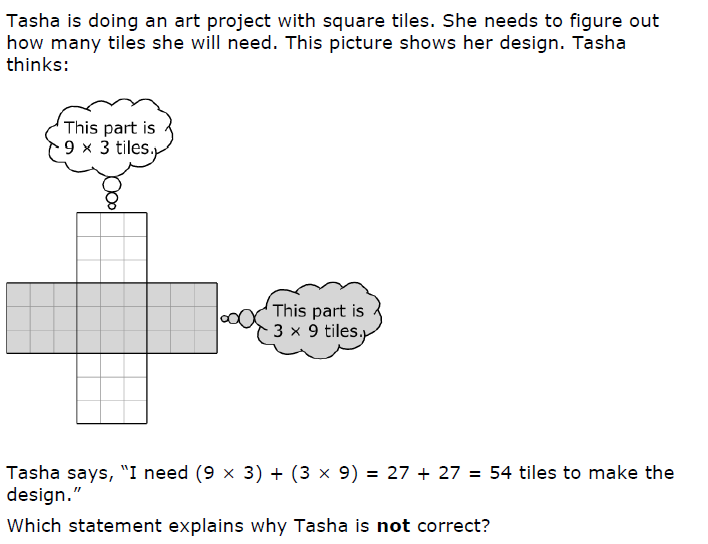12. *
1 point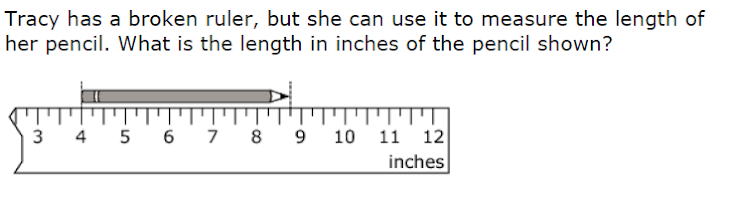13. Select equation(s) that can be used to find the volume.
1 point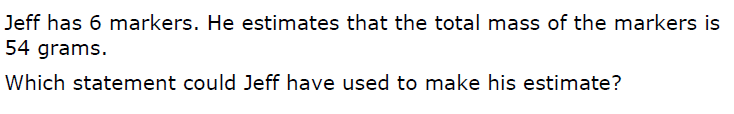14. *
1 point